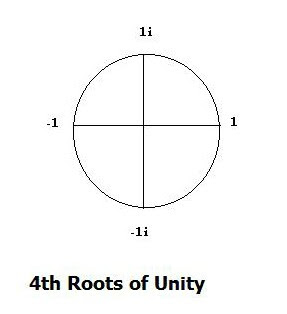Monday, May 1, 2017

Math Revisited: Complex Analysis (2) Complex Roots

Complex Roots:

If z = r cis(
q) is a complex number different from 0, and n is a positive integer, then there are exactly n different complex numbers: w1, w2, w3……wn each of which is the nth ROOT of z. If we let w = r cis(q) be an nth root of z = rcis(q) so wn = z

It can eventually be shown ( I leave this to readers) that:

(r cis(
q))1/n = (r)1/n cis(q/n + k (2 p)/n) ,  with k = 0, +1, +2…..

Problem: take the 3rd roots of 1.

Here: r = 1

The angle is easily determined using and applying the geometry from Galois extensions, for which the number of roots n, divides a unit circle into n equal segments – starting at r = 1 (
q = 0 or 2p) and with the angles given at the boundaries of the n segments

With reference to a simple diagram, the reader should be able to sketch  the results for n = 3, .i.e. for the 3rd roots of 1.  This yields:

2
p/3 = 120 deg  and  4p/ 3 = 240 deg

For the first root of unity (k =0) so:

w0 =  (cos(0)) = 1

For the 2nd root of unity: (k =+1)

w1 = (cos(2p/3) +isin(2p/3)) = - ½ + i(Ö3/2)

For the 3rd root of unity: (k =+ 2)

w2 =  (cos(4p/ 3) + isin(4p/ 3) = - ½ + i(Ö3/2)

Note that the +/-  signs for the roots w1,2 yield two redundant roots. Eliminating them (e.g. removing the secondary sign root duplicates in each case) we have:

w0 = 1

w1 = - ½ + i((
Ö3/2)

w2 = - ½ - i((
Ö3/2)

Example Problem:

Find the fourth roots of unity and provide a sketch diagram to locate them.

SolutionThe diagram isWe have, n = 4 with:
wn = cos (2 p)k/ n + isin(2 pk)/n
For k = 0, 1, 2 and 3 then,

The first root:: w0 = cos(0) + isin(0) = 1

The second root:  w1 = cos (
p/2) + isin(p/2) = 1i = i

The third root:  w2 = cos (
p) + isin(p) = -1

And the fourth root:

w3 = cos(3 p/2) + isin(3p/2) = -1i = -i

All of which can be checked using the accompanying diagram which is part of the problem solution.
Note the roots correspond to successive increases of the angle by p/2 = 90 degrees: